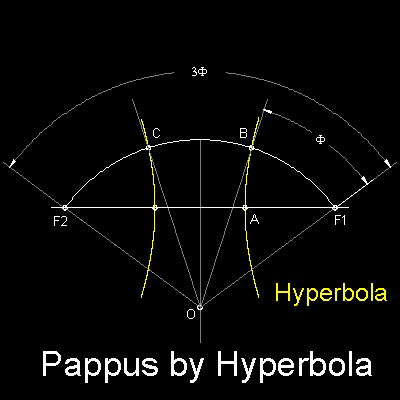Special Curves
Go to   Fun_Math Content Table   Trisecting an Angle   Special Curves

### Trisection using Special Curves

#### The Hyperbola-Pappus

Pappus (280 - 350) showed that "Hyperbola" can be used for Angle Trisection. His idea is shown in the figure shown below.The general formula for this hyperbola is given by

y2 + (1-e2)x2-2kx + k2 = 0

where k = distance of F1 to directrix (X-coordinate value of point F1) =half the distance between B & C.

and e = eccentricity = 2 in this case.
In general, e> 1 for hyperbola

You can see the process in animation.

For detail, go to the section on Conics.
****** Pappus_hyperbola_tri_desc.dwg ******

To create this drawing and animation: